# Exam-Style Questions.

## Problems adapted from questions set for previous Mathematics exams.

### 1.

IGCSE Core

A bricklayer estimates the number of bricks he will need to build a wall by dividing the area of the wall by the area of the face of a brick.

The wall is 16 metres long and 1.2 metres tall.

The bricks he will use are each 25 centimetres long and 12 centimetres tall.

Calculate an estimate for the number of bricks the bricklayer will need to build the wall.

The diagram above of part of the wall is not drawn to scale.

### 2.

GCSE Higher

The diagram shows a water tank in the shape of a cylinder. It has a diameter of 76cm anf a height of 36cm.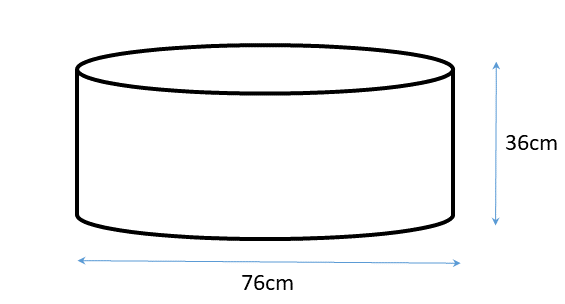It is filled at the rate of 0.3 litres per second. How long does it take to completely fill the tank?

[1 litre = 1000 cm3]

### 3.

GCSE Higher

Babatunde has to paint four containers.

Each container is in the shape of a cylinder with a diameter of 1.2m and a length of 3.2m.

How many tins of paint will Babatunde need to buy to completely cover each of the four containers if each tin of paint covers 6m2?

You must show all of your working.[The surface area of a cylinder of radius $$r$$ and height (or length) $$h$$ is $$2\pi rh + 2\pi r^2$$]

### 4.

GCSE Higher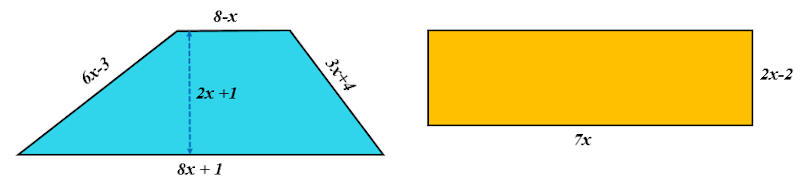The trapezium and rectangle shown here have the same perimeters. The diagrams are not drawn to scale and the measurements are in centimetres. Calculate the area of the trapezium.

### 5.

GCSE Higher

The diagram below shows two rectangles not drawn to scale.

The perimeter of rectangle EFGH is 41cm and the area of rectangle ABCD is 55cm2.

• EF = 9.5cm
• AB = FG### 6.

GCSE Higher

A container is in the shape of a cuboid as shown in the diagram below.

The container is three quarters full of water.

A glass holds 250 ml of water.

What is the greatest number of glasses that can be completely filled with water from the container?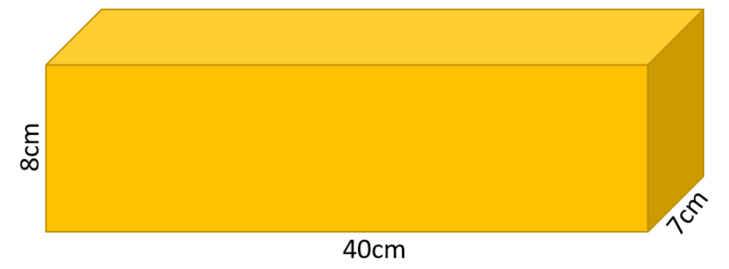### 7.

GCSE Higher

A square has sides of length $$x$$ cm.

The equilateral triangle next to it has sides which are each 3cm more than the length of a side of the square.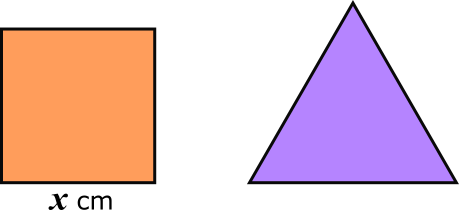(a) Find the perimeter of the square if it is equal to the perimeter of the triangle.The diagram above show the same square and triangle.

The length of the diagonal of the square is $$d$$ cm and the height of the triangle is $$h$$ cm.

(b) Which has the greater value, $$d$$ or $$h$$?

### 8.

GCSE Higher

The diagram shows a water tank in the shape of a trapezoidal prism.Winthrop begins filling the tank with a hose pipe. After 30 minutes there are 900 litres of water in the tank. How many more minutes will it take until the tank is half full? ( $$1m^3 = 1000$$ litres )

### 9.

IB Applications and Interpretation

A wedge is to be cut from a log in the shape of a cylinder as shown in the diagram below (not to scale).

The length of the log is 240cm and its radius is 40cm. The cross section of the wedge to be removed is a sector with an angle of 130o.

What is the volume of the remaining piece of the log after the wedge has been removed?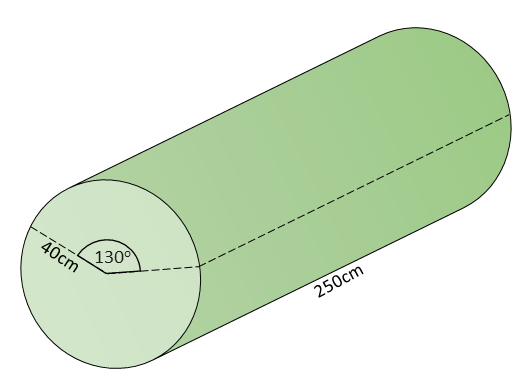If you would like space on the right of the question to write out the solution try this Thinning Feature. It will collapse the text into the left half of your screen but large diagrams will remain unchanged.

The exam-style questions appearing on this site are based on those set in previous examinations (or sample assessment papers for future examinations) by the major examination boards. The wording, diagrams and figures used in these questions have been changed from the originals so that students can have fresh, relevant problem solving practice even if they have previously worked through the related exam paper.

The solutions to the questions on this website are only available to those who have a Transum Subscription.

Exam-Style Questions Main Page

Search for exam-style questions containing a particular word or phrase:

To search the entire Transum website use the search box in the grey area below.